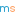Were you a Choco Leibniz or a Fig Newton?This post contains the answer to these puzzles. If you haven’t tried them yet, have a go now!

### An Integral

Let’s name the two integrals:
$$I=\int_0^{\frac\pi2}\frac1{1+\tan^ax}\,dx$$
$$J=\int_0^{\frac\pi2}\frac1{1+\cot^ax}\,dx$$
Replacing $\tan$ with $\sin/\cos$ and a bit of simplifying gives:
$$I=\int_0^{\frac\pi2}\frac{\cos^ax}{\cos^ax+\sin^ax}\,dx$$
$$J=\int_0^{\frac\pi2}\frac{\sin^ax}{\cos^ax+\sin^ax}\,dx$$
Now let’s look at $I+J$:
$$I+J=\int_0^{\frac\pi2}\frac{\cos^ax}{\cos^ax+\sin^ax}\,dx+\int_0^{\frac\pi2}\frac{\sin^ax}{\cos^ax+\sin^ax}\,dx$$
$$=\int_0^{\frac\pi2}\frac{\cos^ax+\sin^ax}{\cos^ax+\sin^ax}\,dx$$
$$=\int_0^{\frac\pi2}1\,dx$$
$$=\frac\pi2$$

By making the substution $u=\frac\pi4-x$, it can be shown that $I=J$. Therefore, $I=\frac\pi4$.

Incredibly, the solution of this puzzle is a constant, even though at first glance the integral looks to depend on $a$.

### $x$ to the power of $x$ to the power of $x$ to the power of …

Let $\displaystyle y=x^{x^{x^{x^{.^{.^.}}}}}$. As the chain of $x$s goes on forever, we can write this as $y=x^y$ or $y=e^{y\ln x}$. Differentiating this gives:
$$\frac{dy}{dx} = e^{y\ln x}\frac{d}{dx}(y\ln x)\\ = e^{y\ln x}\left(\frac{y}x + \ln x\frac{dy}{dx}\right)$$
Rearranging gives:
$$(1+e^{y\ln x}\ln x)\frac{dy}{dx} = \frac{ye^{x\ln y}}x$$
$$\frac{dy}{dx} = \frac{ye^{x\ln y}}{x(1+e^{y\ln x}\ln x)}\\ = \frac{yx^y}{x(1+x^y\ln x)}\\ = \frac{y^2}{x(1+y\ln x)}$$

### Differentiate this

First, simplify $\displaystyle y=e^{x^{\frac{\ln(\ln x)}{\ln x}}}$.
$$y =e^{x^{\frac{\ln(\ln x)}{\ln x}}}\\ =e^{e^{\frac{\ln(\ln x)\ln x}{\ln x}}}\\ =e^{e^{\ln(\ln x)}}\\ =e^{\ln x)}\\ =x$$
The derivative of this is 1.

### Find Them All

$$0=\alpha^2-2\alpha^2+\alpha^2\\ =\int_0^1 x^2f(x) dx-2\int_0^1 \alpha xf(x) dx+\int_0^1 \alpha^2f(x)dx\\ =\int_0^1 (x^2-2\alpha x+\alpha^2)f(x)dx\\ =\int_0^1 (x-\alpha)^2 f(x)dx$$
$f(x)$ and $(x-\alpha)^2$ are both positive so this is only possible if one of them if always zero.

But $(x-\alpha)^2$ is only zero when $x=\alpha$; and $\int_0^1 f(x) dx=1$ so $f(x)$ cannot always be zero.

Therefore no such function exists.

Alex Bolton suggested an alternative, possibly neater, way to solve this puzzle.

The first integral, suggests that $f$ is a probability density function on $[0,1]$. Let $X$ be a random variable modelled by $f$.

The second integral shows that the mean of $X$ is $\alpha$, and the second and third integrals together show that the variance of $X$ is 0. This is only possible if $f$ is the Dirac delta “function” at $\alpha$. But this is not continuous, so no such $f$ exists.Matthew Scroggs is a PhD student at UCL working on finite and boundary element methods. His website, mscroggs.co.uk, is full of maths and now features a video of him completing a level of Pac-Man optimally.
@mscroggsmscroggs.co.uk    + More articles by Matthew